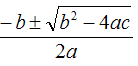# Turbo Pascal 7.0 GuideThe If Statement

## The If Statement - Simple Form

IF expression THEN statement;

The following relational operators can be used to create expressions that evaluate to true or false.

 < is less than > is greater than = is equal to <= is less than or equal to >= is greater than or equal to <> is not equal to

## Example

```PROGRAM scoring; VAR score: integer; BEGIN    write('Input the score');    readln(score);    IF score>60 THEN score:= score +10;    writeln('The final score is ',score);    readln; END.```

A bonus 10 points is added to any score over 60.

## The If Statement - Block If

Sometimes you want a program to do execute more than one line of code if a particular condition is true. In the following program, overtime is added to an employee's wage if they work more than 40 hours in the week.

```PROGRAM payme; VAR hours, overtime : integer; VAR pay : real; BEGIN    write ('Input the number of hours worked ');    readln(hours);    pay:= hours * 3.75;    IF hours>40 THEN    BEGIN       overtime:= hours -40;       pay:= pay + overtime * 3.75;       END;    writeln('Total pay due is £', pay:6:2);    readln; END.```

## The If Statement - Else

In the example program, 3 test scores are entered. The average is calculated and output to the screen. If the average is 55 or more, a pass grade is achieved - otherwise a fail is reported.

```PROGRAM results; VAR score1, score2, score3: integer; VAR average: real; BEGIN    writeln('Input the scores for the three tests ');    write('Test 1 ');    readln(score1);    write('Test 2 ');    readln(score2);    write('Test 3 ');    readln(score3);    average:= (score1+score2+score3)/3;    writeln('Average Test Score is :', average:5:2);    IF average>=55 THEN writeln('Pass') ELSE writeln('Fail');    readln; END.```

## The If Statement - ElseIf

The elseif statement can be used to allow you to check several conditions within one if statement.

```PROGRAM results; VAR score1, score2, score3: integer; VAR average: real; BEGIN    writeln('Input the scores for the three tests ');    write('Test 1 ');    readln(score1);    write('Test 2 ');    readln(score2);    write('Test 3 ');    readln(score3);    average:= (score1+score2+score3)/3;    writeln('Average Test Score is :', average:5:2);    IF average>=75 THEN writeln('Grade A')    ELSE IF average>=65 THEN writeln('Grade B')    ELSE IF average>=55 THEN writeln ('Grade C')    ELSE writeln('Fail');    readln; END. Notice the way that the semicolon only appears after the last part of the if statement. Once the condition has been satisfied, the rest of the if statement is not examined. Exercises Write a program that reads an employee's number, their hourly rate and the number of hours worked in that week. Hours over 40 are paid at time and a half. Calculate and display the wages due to this person. Write a program that reads two numbers and examines the relationship between them. Output one of the following messages,A is greater than BB is greater than AA and B are equal Write a program to find the two roots of the quadratic equation ax2 + bx + c = 0 using the formula,The user inputs values for a, b and c. If b2 - 4ac is negative, then the equation has no roots. If b2 - 4ac=0, the equation has only one root ```
``` ```
``` Pages designed and coded by MHA since 2003 | Valid HTML 4.01(Strict) | CSS ```
``` ```
``` ```